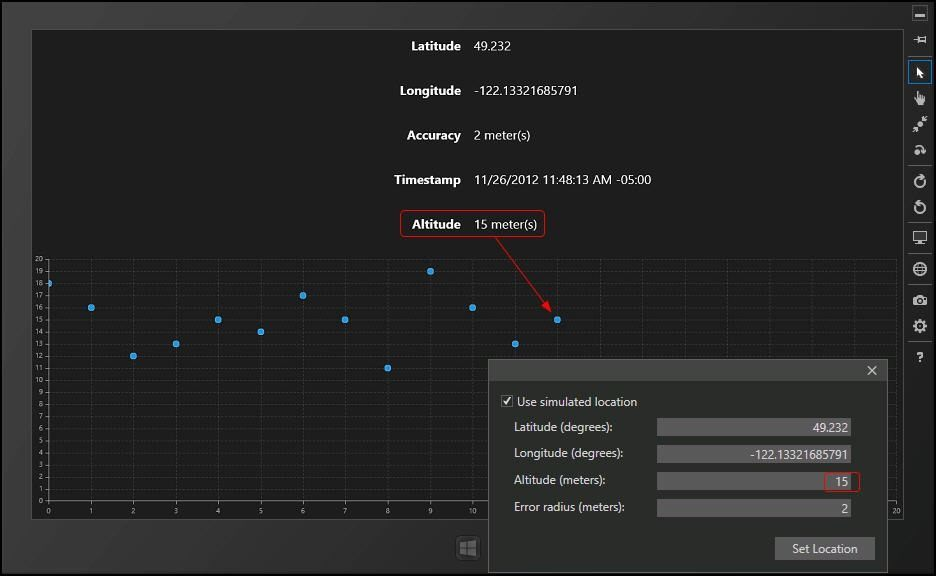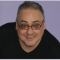In this posting, we’ll look at how to track location coordinates in Windows 8 and how to plot changes in position using Telerik chart controls.  Specifically, we’ll chart changes in altitude (e.g., of a toy helicopter) over time, as shown in figure 1.Figure 1

In Figure 1 we see the current altitude (at 12 minutes) is 15 meters. This is reflected in the scatter diagram and is also reflected in the simulated location information that we’re using to simulate the flight of our simulated helicopter.

# Setting Up

In order to get this to work, we need to be able to obtain the values we’re displaying (e.g., for Latitude and Longitude) and we need to be able to plot a series of values (e.g., for Altitude) against our scatter plot diagram.  Let’s start by looking at how we can get the location values.

To get set up, we’ll create a new Windows 8 Blank application in C# and XAML.  Double click on the Package.appxmanifest and click on the Capabilities tab.  There we’ll want to check the Location box to allow use of location services.  The user still must give permission when the application runs, but this is taken care of for you by the operating system.

We begin in MainPage.xaml by setting up textblocks; some to act as labels and others to hold the values we’ll retrieve from the location services.  To do this, add six RowDefinitions to your grid, as shown in listing 1.

`<Grid Background="{StaticResource ApplicationPageBackgroundThemeBrush}">`
`<Grid.ColumnDefinitions>`
`    <ColumnDefinition Width="*" />`
`    <ColumnDefinition Width="*" />`
`</Grid.ColumnDefinitions>`
`<Grid.RowDefinitions>`
`    <RowDefinition />`
`    <RowDefinition />`
`    <RowDefinition />`
`    <RowDefinition />`
`    <RowDefinition />`
`    <RowDefinition Height="3*" />`
`</Grid.RowDefinitions>`

Listing 1

All of the TextBlocks will use the Style “Output” shown in listing 2.

`<Style x:Name="Output"`
`       TargetType="TextBlock">`
`    <Setter Property="FontFamily"`
`            Value="Segoe UI" />`
`    <Setter Property="FontSize"`
`            Value="40" />`
`    <Setter Property="Margin"`
`            Value="10" />`
`</Style>`

Listing 2

The labels will identify the values, and the TextBlocks in column 1 (the second column) will display their values (see figure 1),

`<TextBlock Text="Latitude"`
`           Grid.Row="0"`
`           Grid.Column="0"`
`           HorizontalAlignment="Right"`
`           FontWeight="Bold"`
`           Style="{StaticResource Output}" />`
`<TextBlock Name="LatitudeTB"`
`           Grid.Row="0"`
`           Grid.Column="1"`
`           HorizontalAlignment="Left"`
`           Style="{StaticResource Output}" />`
`<TextBlock Text="Longitude"`
`           FontWeight="Bold"`
`           Grid.Row="1"`
`           Grid.Column="0"`
`           HorizontalAlignment="Right"`
`           Style="{StaticResource Output}" />`
`<TextBlock Name="LongitudeTB"`
`           Grid.Row="1"`
`           Grid.Column="1"`
`           HorizontalAlignment="Left"`
`           Style="{StaticResource Output}" />`
`<TextBlock Text="Accuracy"`
`           FontWeight="Bold"`
`           Grid.Row="2"`
`           Grid.Column="0"`
`           HorizontalAlignment="Right"`
`           Style="{StaticResource Output}" />`
`<TextBlock Name="AccuracyTB"`
`           Grid.Row="2"`
`           Grid.Column="1"`
`           HorizontalAlignment="Left"`
`           Style="{StaticResource Output}" />`
`<TextBlock Text="Timestamp"`
`           FontWeight="Bold"`
`           Grid.Row="3"`
`           Grid.Column="0"`
`           HorizontalAlignment="Right"`
`           Style="{StaticResource Output}" />`
`<TextBlock Name="TimestampTB"`
`           Grid.Row="3"`
`           Grid.Column="1"`
`           HorizontalAlignment="Left"`
`           Style="{StaticResource Output}" />`
`<TextBlock Text="Altitude"`
`           FontWeight="Bold"`
`           Grid.Row="4"`
`           Grid.Column="0"`
`           HorizontalAlignment="Right"`
`           Style="{StaticResource Output}" />`
`<TextBlock Name="AltitudeTB"`
`           Grid.Row="4"`
`           Grid.Column="1"`
`           HorizontalAlignment="Left"`
`           Style="{StaticResource Output}" />`

# GeoLocation Data

Before looking at how we add the scatter chart, let’s see how we gather the geolocation data and attach the values to the TextBlocks.  In MainPage.xaml.cs we start by defining an object of type Geolocator,

`private Geolocator location;`

As noted in the Microsoft help page this class supports two events:

• PositionChanged – raised when the location updates
• StatusChanged – raised when the ability of the Geolocator to provide updated locations changes

We will create handlers for the first of these, registering the handlers in when we navigate to the page, and de-registering when we leave the page,

`protected override void OnNavigatedTo(NavigationEventArgs e)`
`{`
`    location = new Geolocator();`
`    location.PositionChanged += location_PositionChanged;`
`}`
`protected override void OnNavigatedFrom( NavigationEventArgs e )`
`{`
` base.OnNavigatedFrom( e );`
`    location.PositionChanged -= location_PositionChanged;`
`}`

# Location Changed

Each time the location changes the PositionChanged event is raised and our handler method is called.  In that event handler, we will asynchronously obtain the data we need to update the position TextBlocks,

`async void location_PositionChanged( Geolocator sender, PositionChangedEventArgs args )`
`{`
`    await Dispatcher.RunAsync( CoreDispatcherPriority.Normal, () =>`
`    {`
`        Geoposition position = args.Position;`
`        LatitudeTB.Text = position.Coordinate.Latitude.ToString();`
`        LongitudeTB.Text = position.Coordinate.Longitude.ToString();`
`        AccuracyTB.Text = position.Coordinate.Accuracy.ToString() + " meter(s)";`
`        TimestampTB.Text = position.Coordinate.Timestamp.ToString();`
`        AltitudeTB.Text = position.Coordinate.Altitude.ToString() + " meter(s)";`
`    } );`
`}`

# Plotting the Data

While text data is interesting, nothing is as compelling as a well-designed chart.  To plot the position of our simulated helicopter, we’ll add a Telerik RadCartesianChart – specifically a scatter diagram, to our display.  Returning to MainPage.xaml we’ll add the following,

`<telerik:RadCartesianChart PaletteName="DefaultDark"`
`                           x:Name="xScatterChart"`
`                           Grid.Row="7"`
`                           Grid.Column="0"`
`                           Grid.ColumnSpan="2">`
`    <telerik:RadCartesianChart.HorizontalAxis>`
`        <telerik:LinearAxis Minimum="0"`
`                            MajorStep="1"`
`                            Maximum="20" />`
`    </telerik:RadCartesianChart.HorizontalAxis>`
`    <telerik:RadCartesianChart.VerticalAxis>`
`        <telerik:LinearAxis Minimum="0"`
`                            Maximum="20"`
`                            MajorStep="1" />`
`    </telerik:RadCartesianChart.VerticalAxis>`
`    <telerik:RadCartesianChart.Grid>`
`        <telerik:CartesianChartGrid MajorLinesVisibility="XY" />`
`    </telerik:RadCartesianChart.Grid>`
`    <telerik:ScatterPointSeries ItemsSource="{Binding Altitudes}">`
`        <telerik:ScatterPointSeries.XValueBinding>`
`            <telerik:PropertyNameDataPointBinding PropertyName="Minutes" />`
`        </telerik:ScatterPointSeries.XValueBinding>`
`        <telerik:ScatterPointSeries.YValueBinding>`
`            <telerik:PropertyNameDataPointBinding PropertyName="Height" />`
`        </telerik:ScatterPointSeries.YValueBinding>`
`    </telerik:ScatterPointSeries>`
`</telerik:RadCartesianChart>`

The initial part of the definition describes the name and position of the Chart.  We then declare the Horizontal Axis to be of type LinearAxis and set its Minimum value to 0, its Maximum value to 20 and its step to 1.  We do the same for the Linear Axis.  The values on the Y axis represent height in meters, the values on the X axis represent elapsed minutes.

We’ll return to the binding in just a moment, but let’s first create the custom class we need to map onto this chart: the Altitude class,

`public class Altitude`
`{`
` public int Minutes { get; set; }`
` public double Height { get; set; }`
`}`
As you can imagine, we’ll create an Altitude object for each minute of flight, filling in which minute we are tracking in the Minutes property and recording the current height in the Height property.

Normally, I’d use a ViewModel for binding the collection of Altitude objects, but this time I decided to simplify and place these values into the code behind of MainPage.xaml.cs.  We start by creating an ObservableCollection of Altitude objects, and a property for this collection to which the chart can bind,

`private ObservableCollection<Altitude> altitudes = new ObservableCollection<Altitude>();`
`public ObservableCollection<Altitude> Altitudes`
`{`
`    get { return altitudes; }`
`}`

We then extend location_PositionChanged to create a new Altitude object each time the position changes (note that we are assuming one minute between each change, again for simplicity) and we add that Altitude object to our collection,

`if ( position.Coordinate.Altitude != null )`
`{`
`    var alt = new Altitude()`
`    {`
`        Minutes = ctr++,`
`        Height = ( double )position.Coordinate.Altitude`
` `
`    };`
`    altitudes.Add( alt );`

Remember to return to OnNavigatedTo and set the DataContext for the scatter chart to the MainPage object itself,

`xScatterChart.DataContext = this;`

This allows the chart to bind to the Altitudes property.

Returning to the second half of the RadCartesianChart defined in MainPage.xaml (and shown above) we see that the ItemsSource is bound to the Altitudes property and that the XValue is bound to Minutes and the YValue to Height, just as we want and expect.

In this example, we’re using the simulator to change the altitude and otherwise simulate the flight, but the principles remain the same and if you were to run this in a laptop that has a GPS you should receive readings as you raise and lower the laptop.